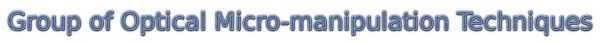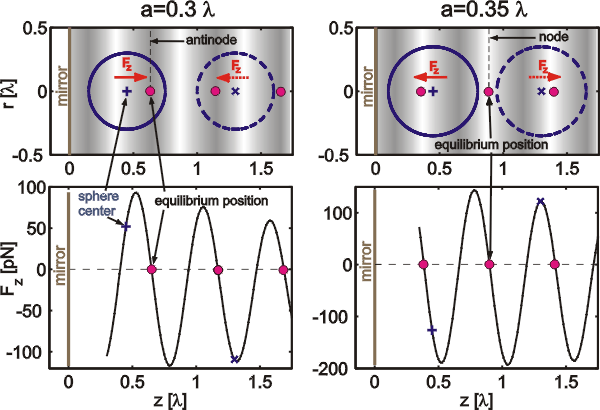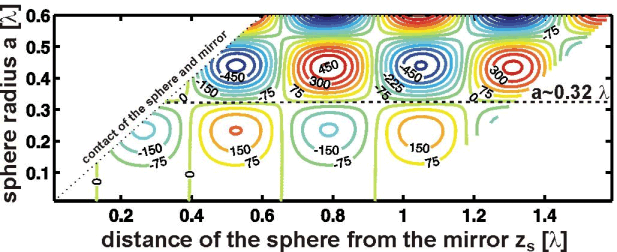OMITEC: Properties of Gaussian standing wave traps

### Properties of Gaussian standing wave traps

If the object placed to the Gaussian standing wave (GSW) is much smaller than the trapping wavelength, the object is pushed to the GSW intensity maxima. However, if the size of the object increases and starts to overlap the neighboring intensity maximum the trapping properties change. This effect is illustrated in the following figure, where two pairs of polystyrene spheres of slightly different radii (a=0.3 l, 0.35 l) are placed symmetrically with respect to the intensity maximum (the sphere centers are depicted by "+" and "x" and their surfaces by full and dashed circles, respectively). While the smaller one is still pushed by axial optical force Fz to the intensity maximum (dark fringe), the center of the bigger one is to the intensity minimum. Therefore their equilibrium positions differ by l/4. Because the axial force changed its sign (see the bottom subplot), there has to exist an object size that is not influenced by the standing wave (axial force is equal to zero).Results of more detailed calculations using the generalized Lorenz-Mie theory (GLMT) are shown in the figure below. We assumed a polystyrene spheres of radii from 0.01l to 0.6l and placed at various positions with respect to the reflecting mirror - standing wave. Red (blue) contours show the positive (negative) axial force acting on the sphere. Green contours correspond to zero force. Black dashed line denotes the sphere size equal toabout 0.32 which is not influenced by the standing wave. This size cannot be optically confined axially in the standing wave.Further comparison of the properties of Gaussian standing wave and single beam traps can be found here or in the related publications.

Related publications

P. Jakl, M. Sery, J. Jezek, A. Jonas, M. Liska, P. Zemanek: "Behaviour of an optically trapped probe approaching a dielectric interface",

P. Zemanek, A. Jonas, P. Jakl, J. Jezek, M. Sery, M. Liska: "Theoretical comparison of optical traps created by standing wave and single beam",